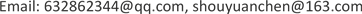1北京师范大学天文系，北京

2山东师范大学，信息科学与工程学院，山东 济南1. 引言

2. 宇宙大爆炸论的天文观测依据

1929年，哈勃利用当时全世界口径最大的光学望远镜(口径：2.54米)，测量到河外遥远星系，发来的光，其谱线有向红的一点移动的现象(就是频率降低)，不认为是一个全新的物理规律。沿用1846年多普勒发现的效应，波源移动，引起波长变化，用其解释测量现象，得到星系后退，后退速度与星系距离成比例的关系式，被称为哈勃定律。

1964年，彭齐亚斯和威尔逊在巨型号角天线上，测量到2.7的微波背景辐射，被定义为是宇宙大爆炸的余辉，获得1978诺贝尔物理奖；1989年11月，约翰·马瑟、乔治·斯穆特主持升空COBE卫星，测量到符合温度为2.726 K的黑体辐射谱的背景辐射，再一次认定为大爆炸余辉，并获得2006诺贝尔物理奖。

1999年，索尔·波尔穆特、布莱恩·施密特和亚当·里斯研究超新星，发现亮度衰减更快、红移更大，推理出宇宙加速膨胀的结论，并获得2011诺贝尔物理奖。

3. 陈寿元效应3.1. 定义

3.2. 陈寿元效应的理论论述

∅ B − ∅ A = − E ( B − A ) (1)

(1)式中：E——能场强度，克服能量耗散而做功。波动从A点传递到B点的必要条件： ∅ A > ∅ B .

A点的能场强度 E A ：

E A = − lim B → A ( ∅ B − ∅ A B − A ) (2)

∅ ( r ) = Q 4   ∈   π ( r − r ′ ) (3)

E——能场强度：可用能位函数的负梯度来表示：

E = − ∇ ∅ (4)

3.2.1. 质点的无阻尼自由振动

y = A cos ( ω t + θ ) (5)

u = d y d t = − A ω sin ( ω t + θ ) (6)

α = d 2 x d t 2 = − ω 2 A cos ( ω t + θ ) (7)

E k = 1 2 m υ 2 = 1 2 m A 2 ω 2 sin 2 ( ω t + θ ) (8)

∅ k = E K m = 1 2 ω 2 A 2 sin 2 ( ω t + θ ) (9)

(8)和(9)式表明质点的振动能 E K 、振动能位函数与振动的频率平方成正比。

3.2.2. 振荡电偶极子产生的电磁波能

p = g L = g l cos ω t (10)

E θ = 1 4 π ε 0 P 0 ω 2 sin θ C 2 r cos ( ω t − k r ) (11)

B op = μ 0 4 π P 0 ω 2 sin θ C r cos ( ω t − k r ) (12)

(11)、(12)式表明，振荡电偶极子在远处产生电场、磁场强度与振荡频率平方成正比。

S = E × H = P 0 2 ω 4 sin 2 θ 16 π 2 ε 0 C 3 r 2 ( cos ( ω t − k r ) ) 2 (13)

(13)式表明电偶极子辐射电磁波的能流密度与偶极子振荡频率四次方成正比。

3.2.3. 波动的能位函数

Y ( x , t ) = A ( x ) cos ( ω ( x ) t − k x ) (14)

(14)式中：A(x)——波的振幅，通常随传播距离而衰减，是X的函数。 ω ( x ) 角速度，目前认为它不随传播距离变化，是不变量。但是前面的分析，振源能量与频率平方成正比。能量是要消耗，扩散、色散。能量在空间上的散开，表现占用更大面积或更大的体积空间，使波长变长。在时域上，能量散开意味着占用更多的时间段，使振动的周期有延长的趋势。

υ y = ∂ Y ( x , t ) ∂ t = − A ( X ) ω ( X ) sin ( ω t − k x ) (15)

op y ( x , t ) = 1 2 υ y 2 = 1 2 A 2 ( x ) ω 2 ( x ) ( sin ( ω t − k x ) ) 2 (16)

(16)式表明波动的能位函数与波动的频率平方成正比。

op ( x ) = 1 4 A 2 ( x ) ω 2 ( x ) (17)

d p d x = − α p

p ( x ) = p ( 0 ) e − α x (18)

dop d x = − α op

op ( x ) = op ( 0 ) e − α x (19)

A 2 ( x ) ω 2 ( x ) = A 2 ( 0 ) ω 2 ( 0 ) e − α x (20)

A ( x ) ω ( x ) = A ( 0 ) ω ( 0 ) e − 1 2 α x (21)

1) 若信号传输过程中，频率不变，即：

ω ( x ) = ω (0)

2) 若信号传输过程中，振幅保持不变，即：

A ( x ) = A (0)

3)共同分担信号的衰减量：

A ( x ) = A ( 0 ) e − 1 4 α x (24)

ω ( x ) = ω ( 0 ) e − 1 4 α x (25)

4) A ( x ) < A ( 0 ) e − 1 2 α x (26)

5) ω ( x ) < ω ( 0 ) e − 1 2 α x (27)

6) 更一般现实情况，A(x)衰减快， ω ( x ) 衰减慢。波的形态分三段：波能量密度很大，随传播距离，振幅衰减为主，频率衰减为辅——称为波状态；波能量密度小，振幅衰减为辅，频率衰减为主——量子状态；波能量密度非常小，振幅、频率都快速衰减为零——波消亡态。从 k e − 1 2 α x 到 e − 1 4 α x 之间变化。

3.3. 实验的技术方案3.3.1. 水波观察

3.3.2. 声波实验

3.3.3. 电磁波实验

z = λ − λ e λ e = H 0 c D (28)

D = 2 × 10 6 × 3.086 × 10 13 km = 6.172 × 10 22 m ；

H 0 = 500   km ⋅ s − 1 ⋅ Mpc − 1

Δ λ = λ − λ e = z λ e = H 0 C D λ e (29)

z = λ − λ e λ e = c f − c f e c f e = f e − f f

Δ f = f e − f = z f = H 0 c D f (30)

Δ θ = 360 ( f e − f ) t = 360 H 0 C D f t (2.31)

4. 对宇宙大爆炸论讨论4.1. 宇宙红移的解释

4.2. 超新星的解释

1989年，索尔·波尔穆特、布莱恩·施密特和亚当·里斯研究超新星，发现亮度衰减更快、红移更大，用多普勒效应解释，推理出宇宙加速膨胀的结论，并获得2011诺贝尔物理奖。

4.3. 背景辐射

4.4. 宇宙物质风度

4.5. 宇宙大爆炸与哲学矛盾

4.6. 宇宙大爆炸与物理学矛盾

4.7. 宇宙大爆炸与地心说对比

4.8. 宇宙大爆炸与日心说比较

5. 结论

1) 把宇宙红移现象用多普勒效应来解释，得到宇宙膨胀、宇宙爆炸的结论；用频率衰减来解释，光的红移现象就是光频率在传播过程中，由于波能能量消耗，使频率减小，这将导致波长增长。实验表明，振幅衰减快，频率衰减非常缓慢。在目前技术手段下，难以测量到这种微小变化。通信工程，几万公里的传播范围内，可以认为频率不减小。超精密实验设计可以测量到频率极其微弱的衰减。

2) 宇宙大爆炸论与其他科学矛盾重重，用光信号在太空传播，频率衰减，一切矛盾迎刃而解。